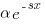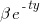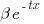## Will They Blend? – a.k.

Last time we saw how we can create new random variables from sets of random variables with given probabilities of observation. To make an observation of such a random variable we randomly select one of its components, according to their probabilities, and make an observation of it. Furthermore, their associated probability density functions, or PDFs, cumulative distribution functions, or CDFs, and characteristic functions, or CFs, are simply sums of the component functions weighted by their probabilities of observation.
Now there is nothing about such distributions, known as mixture distributions, that requires that the components are univariate. Given that copulas are simply multivariate distributions with standard uniformly distributed marginals, being the distributions of each element considered independently of the others, we can use the same technique to create new copulas too.

## Mixing It Up – a.k.

Last year we took a look at basis function interpolation which fits a weighted sum of n independent functions, known as basis functions, through observations of an arbitrary function's values at a set of n points in order to approximate it at unobserved points. In particular, we saw that symmetric probability density functions, or PDFs, make reasonable basis functions for approximating both univariate and multivariate functions.
It is quite tempting, therefore, to use weighted sums of PDFs to construct new PDFs and in this post we shall see how we can use a simple probabilistic argument to do so.

## What’s The Lucky Number? – a.k.

Over the last few months we have been looking at Bernoulli processes which are sequences of Bernoulli trails, being observations of a Bernoulli distributed random variable with a success probability of p. We have seen that the number of failures before the first success follows the geometric distribution and the number of failures before the rth success follows the negative binomial distribution, which are the discrete analogues of the exponential and gamma distributions respectively.
This time we shall take a look at the binomial distribution which governs the number of successes out of n trials and is the discrete version of the Poisson distribution.

## Bad Luck Comes In Ks – a.k.

Lately we have been looking at Bernoulli processes which are sequences of independent experiments, known as Bernoulli trials, whose successes or failures are given by observations of a Bernoulli distributed random variable. Last time we saw that the number of failures before the first success was governed by the geometric distribution which is the discrete analogue of the exponential distribution and, like it, is a memoryless waiting time distribution in the sense that the distribution for the number of failures before the next success is identical no matter how many failures have already occurred whilst we've been waiting.
This time we shall take a look at the distribution of the number of failures before a given number of successes, which is a discrete version of the gamma distribution which defines the probabilities of how long we must wait for multiple exponentially distributed events to occur.

## If At First You Don’t Succeed – a.k.

Last time we took a first look at Bernoulli processes which are formed from a sequence of independent experiments, known as Bernoulli trials, each of which is governed by the Bernoulli distribution with a probability p of success. Since the outcome of one trial has no effect upon the next, such processes are memoryless meaning that the number of trials that we need to perform before getting a success is independent of how many we have already performed whilst waiting for one.
We have already seen that if waiting times for memoryless events with fixed average arrival rates are continuous then they must be exponentially distributed and in this post we shall be looking at the discrete analogue.

## One Thing Or Another – a.k.

Several years ago we took a look at memoryless processes in which the probability that we should wait for any given length of time for an event to occur is independent of how long we have already been waiting. We found that this implied that the waiting time must be exponentially distributed, that the waiting time for several events must be gamma distributed and that the number of events occuring in a unit of time must be Poisson distributed.
These govern continuous memoryless processes in which events can occur at any time but not those in which events can only occur at specified times, such as the roll of a die coming up six, known as Bernoulli processes. Observations of such processes are known as Bernoulli trials and their successes and failures are governed by the Bernoulli distribution, which we shall take a look at in this post.

## One Thing Or Another – a.k.

Several years ago we took a look at memoryless processes in which the probability that we should wait for any given length of time for an event to occur is independent of how long we have already been waiting. We found that this implied that the waiting time must be exponentially distributed, that the waiting time for several events must be gamma distributed and that the number of events occuring in a unit of time must be Poisson distributed.
These govern continuous memoryless processes in which events can occur at any time but not those in which events can only occur at specified times, such as the roll of a die coming up six, known as Bernoulli processes. Observations of such processes are known as Bernoulli trials and their successes and failures are governed by the Bernoulli distribution, which we shall take a look at in this post.

## Slashing The Odds – a.k.

In the previous post we explored the Cauchy distribution, which, having undefined means and standard deviations, is an example of a pathological distribution. We saw that this is because it has a relatively high probability of generating extremely large values which we concluded was a consequence of its standard random variable being equal to the ratio of two independent standard normally distributed random variables, so that the magnitudes of observations of it can be significantly increased by the not particularly unlikely event that observations of the denominator are close to zero.
Whilst we didn't originally derive the Cauchy distribution in this way, there are others, known as ratio distributions, that are explicitly constructed in this manner and in this post we shall take a look at one of them.

## Moments Of Pathological Behaviour – a.k.

Last time we took a look at basis function interpolation with which we approximate functions from their values at given sets of arguments, known as nodes, using weighted sums of distinct functions, known as basis functions. We began by constructing approximations using polynomials before moving on to using bell shaped curves, such as the normal probability density function, centred at the nodes. The latter are particularly useful for approximating multi-dimensional functions, as we saw by using multivariate normal PDFs.
An easy way to create rotationally symmetric functions, known as radial basis functions, is to apply univariate functions that are symmetric about zero to the distance between the interpolation's argument and their associated nodes. PDFs are a rich source of such functions and, in fact, the second bell shaped curve that we considered is related to that of the Cauchy distribution, which has some rather interesting properties.

## Calculating statement execution likelihood

In the following code, how often will the variable `b` be incremented, compared to `a`?

If we assume that the variables `x` and `y` have values drawn from the same distribution, then the condition `(x < y)` will be true 50% of the time (ignoring the situation where both values are equal), i.e., `b` will be incremented half as often as `a`.

```a++;
if (x < y)
{
b++;
if (x < z)
{
c++;
}
}
```

If the value of `z` is drawn from the same distribution as `x` and `y`, how often will `c `be incremented compared to `a`?

The test `(x < y)` reduces the possible values that `x` can take, which means that in the comparison `(x < z)`, the value of `x` is no longer drawn from the same distribution as `z`.

Since we are assuming that `z` and `y` are drawn from the same distribution, there is a 50% chance that `(z < y)`.

If we assume that `(z < y)`, then the values of `x` and `z` are drawn from the same distribution, and in this case there is a 50% change that `(x < z)` is true.

Combining these two cases, we deduce that, given the statement `a++;` is executed, there is a 25% probability that the statement `c++;` is executed.

If the condition `(x < z)` is replaced by `(x > z)`, the expected probability remains unchanged.

If the values of `x`, `y`, and `z` are not drawn from the same distribution, things get complicated.

Let's assume that the probability of particular values of `x` and `y` occurring areand, respectively. The constantsandare needed to ensure that both probabilities sum to one; the exponentsandcontrol the distribution of values. What is the probability that `(x < y)` is true?

Probability theory tells us that, where:is the probability distribution function for(in this case:), andthe the cumulative probability distribution for(in this case:).

Doing the maths gives the probability of `(x < y)` being true as:.

The `(x < z)` case can be similarly derived, and combining everything is just a matter of getting the algebra right; it is left as an exercise to the reader :-)